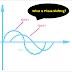In simple words, phase shifting is the procedure to make displacement between two signals respect with to a time domain. Here, basically, the point of the cycles is changed. In other words, it can be said that the difference between phases of two periodic signals is called phase shift, and the procedure of making it is called phase shifting.

Remember that phase shifting does not mean that the two signals have different frequencies. Phase shifting can happen between two signals having the same frequency or different frequencies. Here, the zero-crossing point changes with respect to time. Phase shift is measured in degrees or radians.

The phase shift is also known as phase difference and phase modulation. So when the phase difference is 180 degrees, then the phases will be opposite to each other. So, the phase inversion or phase reversal implies the 180-degree phase shift or phase difference.

Phase-shifting not only happens at 180 degrees, but it can also happen with any degree of angle such as 60 degrees, 90 degrees, 120 degrees, etc.

Now, understand the phase-shifting with an example.

When an AC circuit contains an inductor it causes the lagging power factor. It makes the phase difference between voltage and current waveforms 90 degrees. Here, the current lags behind the voltage by 90 degrees.

When an AC circuit contains a capacitor it causes the leading power factor. It makes the phase shift between the voltage and current 90 degrees leading. Here, the current leads behind the voltage by 90 degrees.

Phase shifting can be done using electronic amplifier circuits, high pass or low pass circuits, etc.

Sometimes the phase-shifting happens automatically due to the internal property of the components of the circuit and sometimes it is done manually. Phase shifting is used for the filtering of signals, demodulation of signals. Sometimes it is used for testing and analyzing.

What is Phase Shifting? Explained with ExamplesReviewed by Author on 1/15/2022 Rating: 5